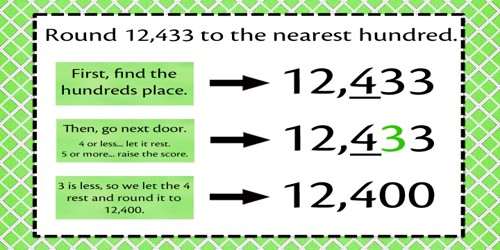Rounding Numbers

• Rounding makes numbers that are easier to work within your head.
• Rounded numbers are only approximate.
• An exact answer generally cannot be obtained using rounded numbers.
• Use rounding to get a answer that is close but that does not have to be exact.

How to round numbers

• Make the numbers that end in 1 through 4 into the next lower number that ends in 0. For example 74 rounded to the nearest ten would be 70.
• Numbers that end in a digit of 5 or more should be rounded up to the next even ten. The number 88 rounded to the nearest ten would be 90.Rounding to the Nearest Hundred

Rounded numbers are easier to work with in your head. Rounded numbers are only approximate. You cannot get an exact answer with rounded numbers. Sometimes an exact answer is non needed.

To round numbers to the nearest hundred, make the numbers that end in 1 through 49 into the next lower number that ends in 00. For example 424 rounded to the nearest hundred would be 400. Numbers that have the last two digits of 50 or more should be rounded up to the next even hundred. The number 988 rounded to the nearest hundred would be 1000.

Rounding to the Nearest Thousand

Rounded numbers are easier to work within your head. They are only approximate. An exact answer cannot be obtained with these numbers. Sometimes an exact answer is not required.

To round numbers to the nearest thousand, make the numbers whose last three digits are 001 through 499 into the next lower number that ends in 000. For example, 6424 rounded to the nearest thousand is 6000. Numbers that have the last three digits of 500 or more should be rounded up to the next even thousand. The number 8788 rounded to the nearest thousand is 9000.

Rounding to the Nearest Ten Thousand

To round numbers to the nearest ten thousand, make the numbers whose last four digits are 0001 through 4999 into the next lower number that ends in 0000. For example 54,424 rounded to the nearest ten thousand would be 50,000. Numbers that have the last four digits of 5000 or more should be rounded up to the next even ten thousand. The number 78,988 rounded to the nearest ten thousand would be 80,000.

Rounding to the Nearest Hundred Thousand

To round numbers to the nearest hundred thousand, make the numbers whose last five digits are 00001 through 49999 into the next lower number that ends in 00000. For example 6,424,985 rounded to the nearest hundred thousand would be 6,400,000. Numbers that have the last five digits of 50000 or more should be rounded up to the next even hundred thousand. The number 8,988,987 rounded to the nearest hundred thousand would be 9,000,000.

Information Source: